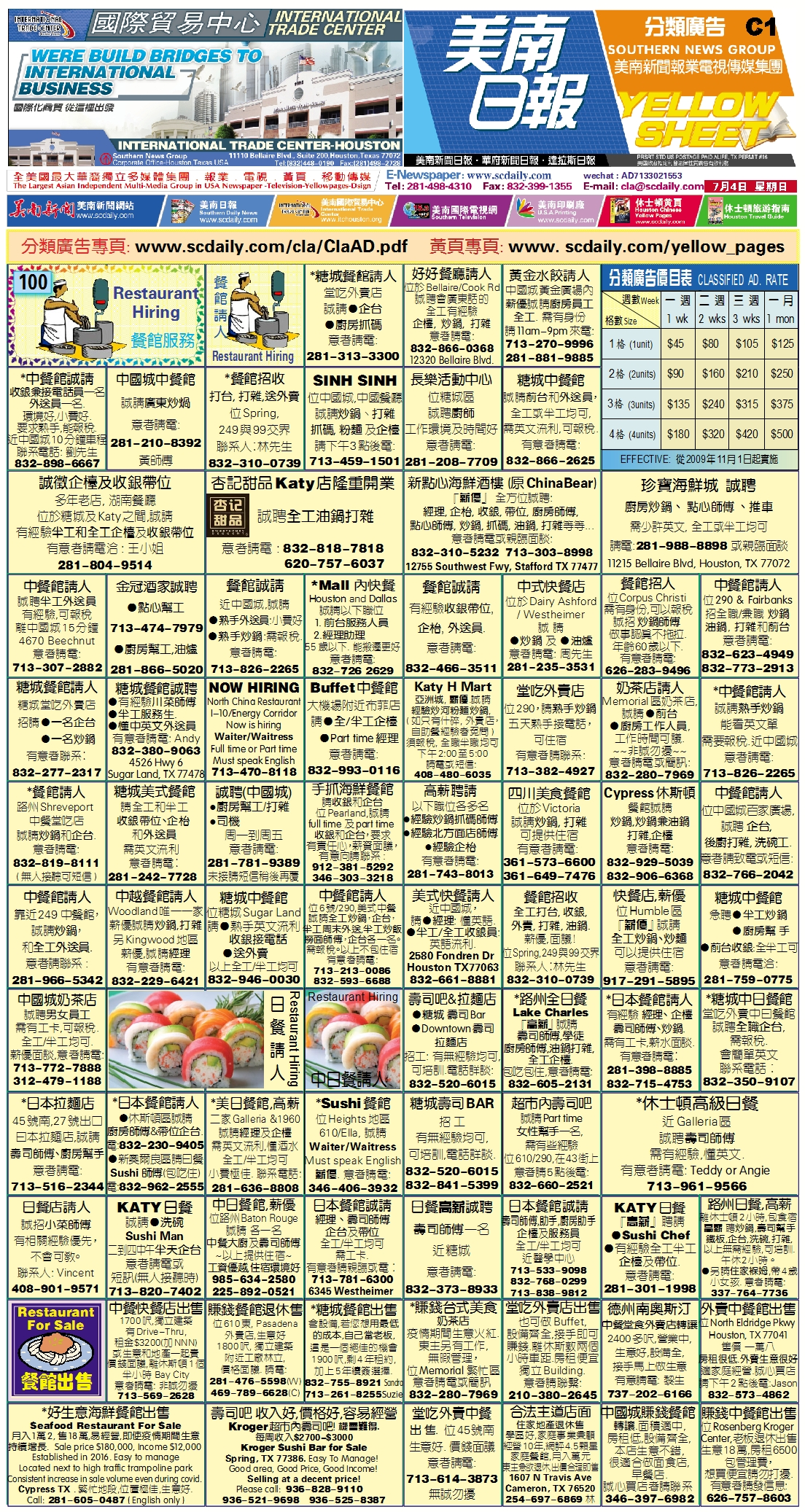210713 Epaper

 A Section B SectionC Section D Section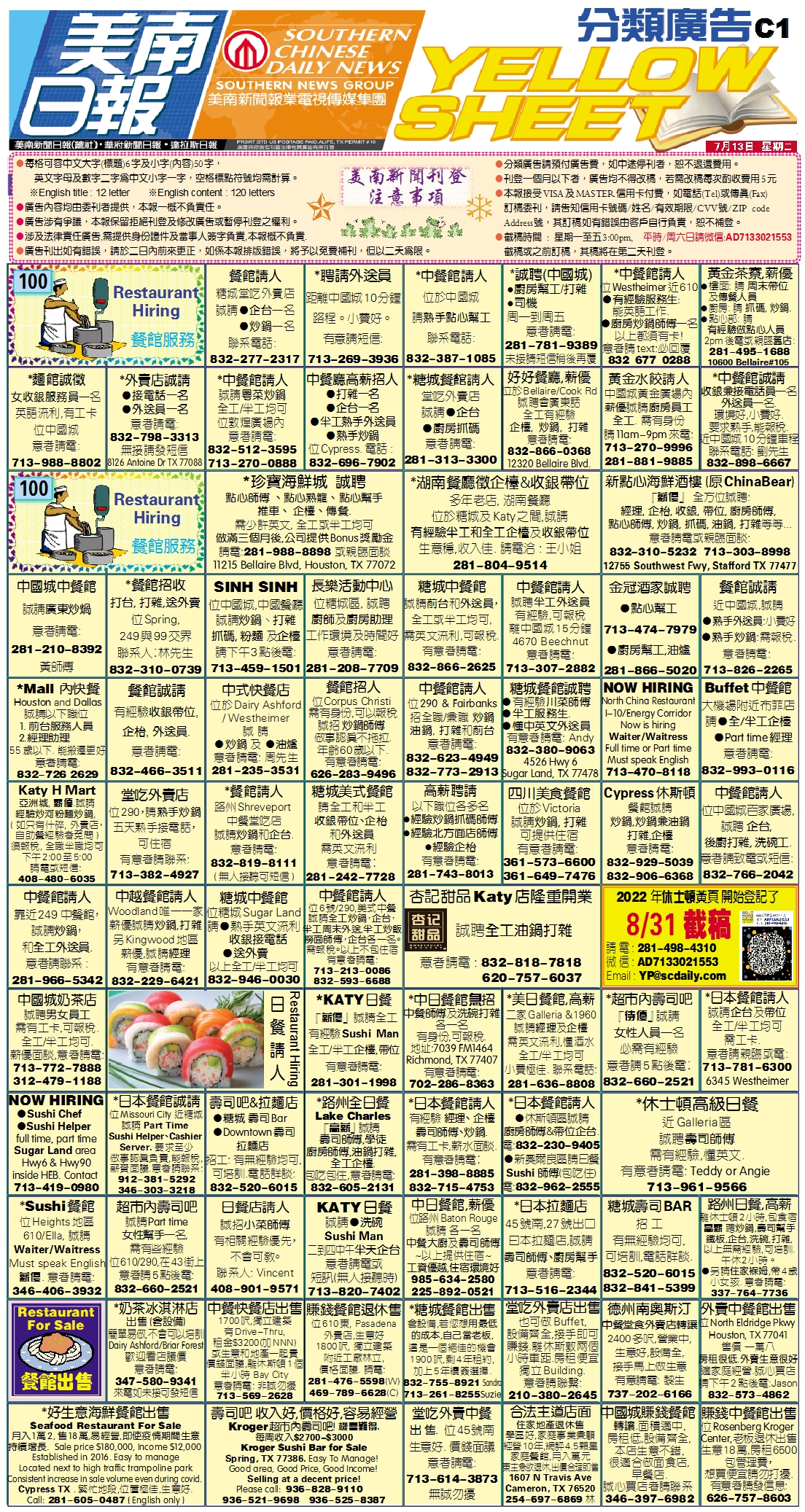210712 Epaper

 A Section B SectionC Section D Section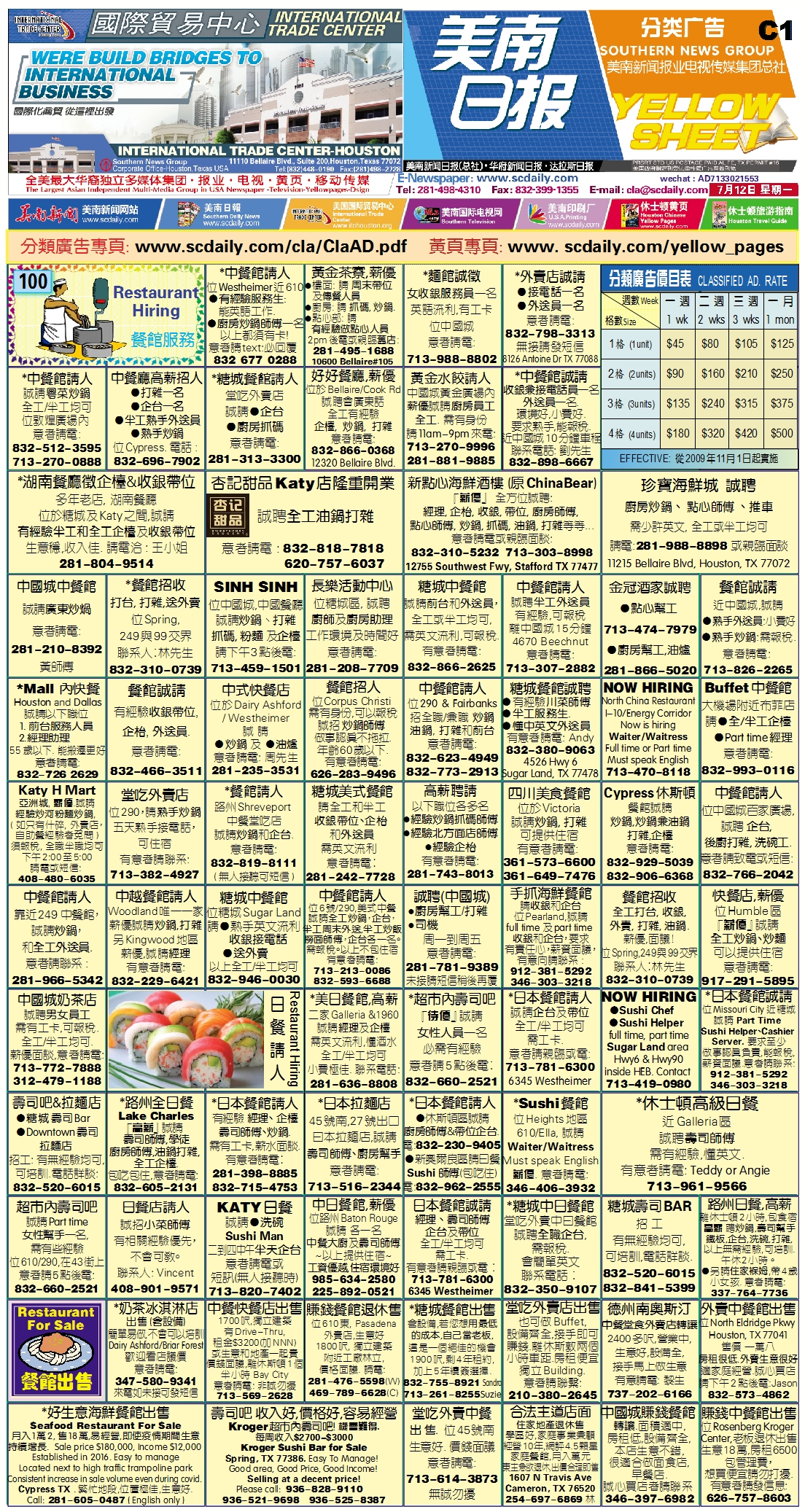210711 Epaper

 A Section B SectionC Section D Section210710 Epaper

 A Section B SectionC Section D Section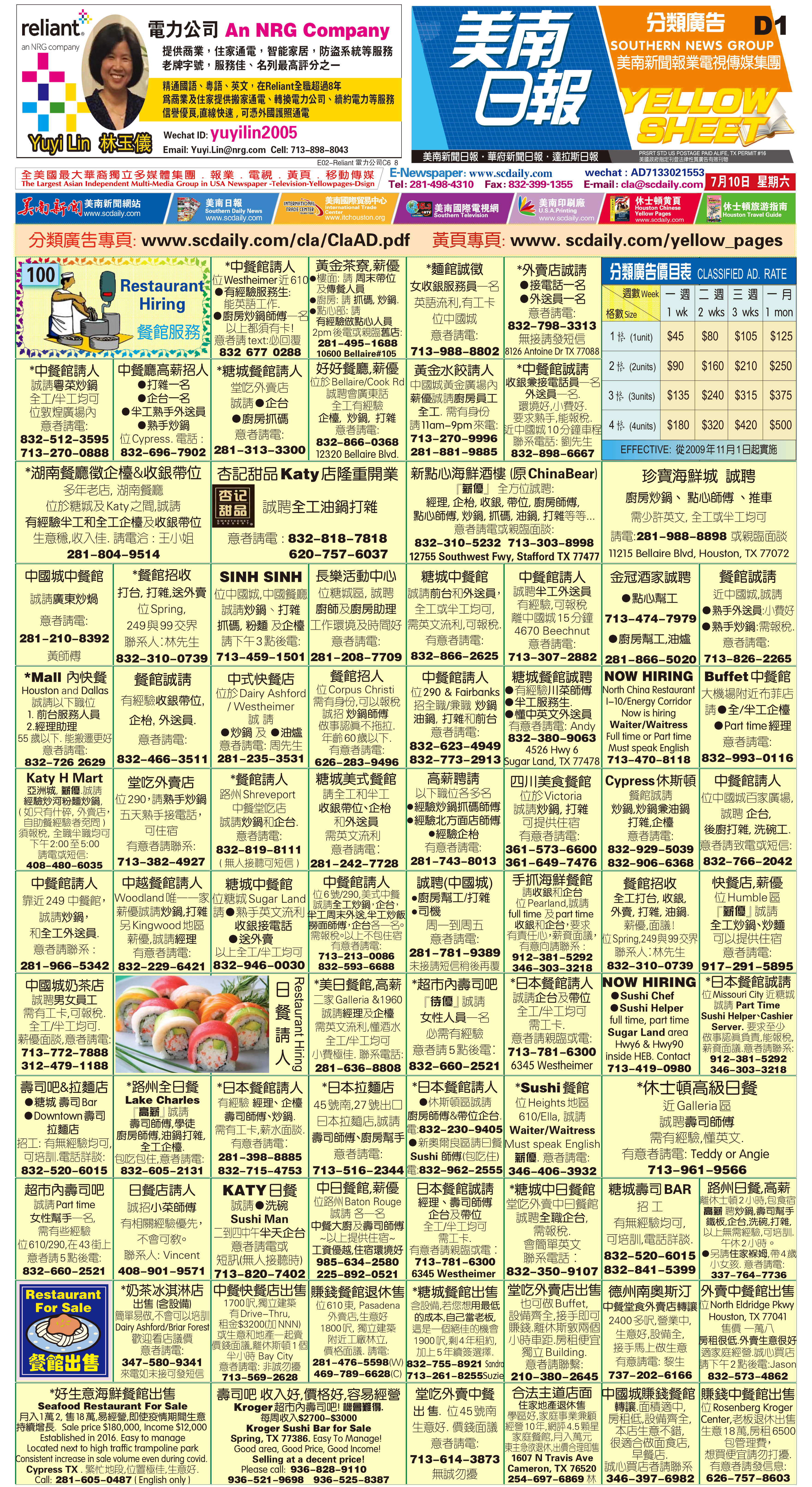210709 Epaper

 A Section B SectionC Section D Section210708 Epaper

 A Section B SectionC Section D Section210707 Epaper

 A Section B SectionC Section D Section210703 Epaper

 A Section B SectionC Section D Section210705 Epaper

 A Section B SectionC Section D Section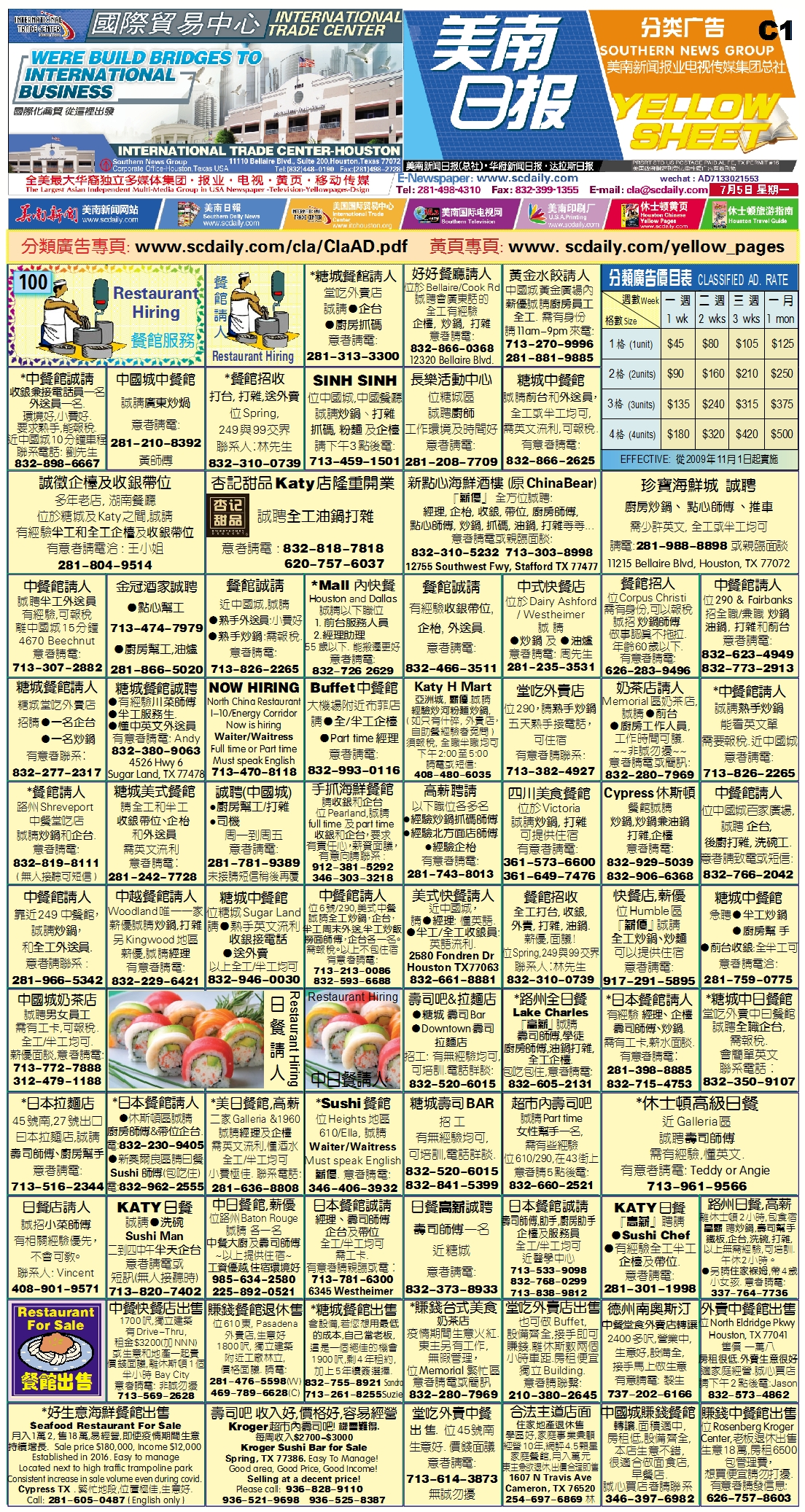210706 Epaper

 A Section B SectionC Section D Section210704 Epaper

 A Section B SectionC Section D Section